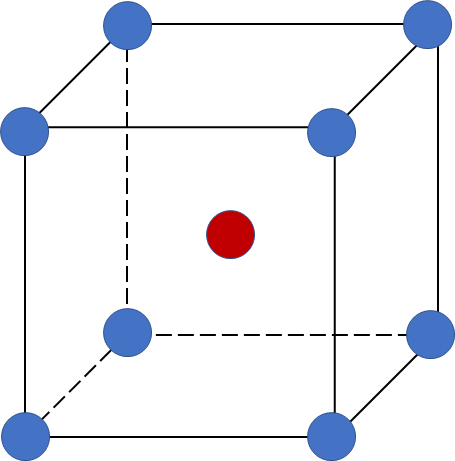# Problem: Barium has a density of 3.59 g/cm3  and crystallizes with a body-centered cubic unit cell. Calculate the radius of a barium atom.

🤓 Based on our data, we think this question is relevant for Professor N/A's class at Ryerson University.

###### FREE Expert Solution

A body-centered cubic (BCC) unit cell is composed of a cube with one atom at each of its corners and one atom at the center of the cubeRecall that the edge length (a) of a BCC unit cell can be calculated using the equation:

$\overline{){\mathbf{a}}{\mathbf{=}}\frac{\mathbf{4}\mathbf{r}}{\sqrt{\mathbf{3}}}}$

Step 1: Calculate the volume of 1 unit cell using density

• molar mass Ba = 137.33 g/mol
• 1 mole = 6.022x1023 entities (Avogadro' number)
entities = atoms, ions, molecules, formula units

recall the # of atoms present per 1 BCC unit cell: corner atoms contribute 1/8 and the center atom contribute 1

# of atoms = 2 per 1 unit cell

Solving volume of 1 unit cell:

volume =1.27x10-22 cm3/1 unit cell###### Problem Details

Barium has a density of 3.59 g/cm3  and crystallizes with a body-centered cubic unit cell. Calculate the radius of a barium atom.